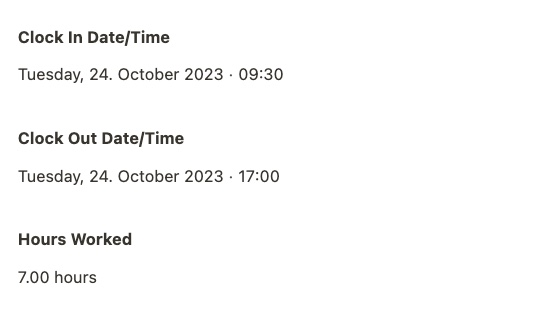# [✅ Solution] How to calculate decimal hours?

I’m creating a timesheet app for a simple punch-in/out. I created a calculation to calculate the hours worked based on clock-in and clock-out times. Here is the calculation:

It is somewhat giving me the correct hour results. However, it ignores the decimals. For example:In the picture above, the hours worked should be 7.50 hours, but the calculation only gives me 7.00 hours.

How can I fix this?

1 Like

Hi @Luis,

you’re on the absolute correct path here - the only issue is that `differenceInHours` will only return the full hours “fitting” into the difference. If you’d like to get accurate decimal hours, you could use `differenceInMinutes` and then divide by 60.

Let me know how it works!

Cheers
Tim

Thanks, @Tim!, for such a simple solution. That worked perfectly. Here is the code for other community members with the same issue:

``````if(﻿@Clock-In Date/Time﻿ && ﻿@Clock-Out Date/Time﻿) {

date_fns.differenceInMinutes(new Date(﻿@Clock Out Date/Time﻿), new Date(﻿@Clock In Date/Time﻿))/60;

} else {

null

}
``````

The if-else is not to show a large number appearing when the clock-out is not set.

4 Likes

… great to hear @Luis and thanks for sharing your final solution.

Happy building
Tim

@Luis here is my little code in similar context where you get only working days from Monday - Friday, exclude weekends so you can get how many working days is within month…

``````var i,day,
start = ﻿@In﻿,
end = ﻿@Out﻿,
dur = moment(end).diff(start,"d")+1,
count = 0;
for(i = 0; i < dur; i++){